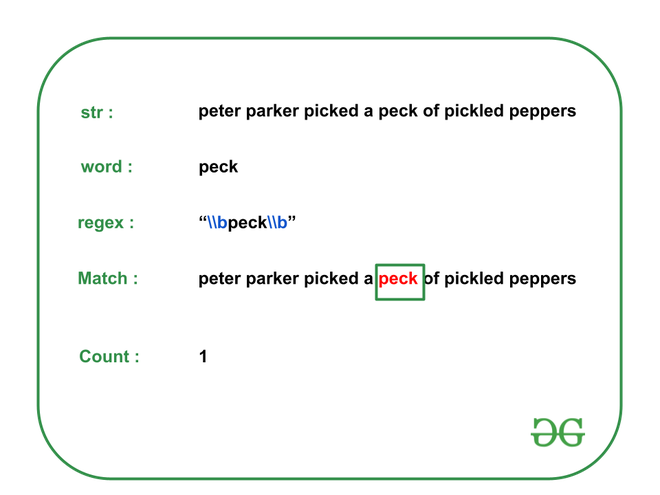Open in App
Not now

# Count occurrences of a word in string | Set 2 (Using Regular Expressions)

• Last Updated : 15 Feb, 2023

Given a string str and a word w, the task is to print the number of the occurrence of the given word in the string str using Regular Expression.

Examples:

Input: str = “peter parker picked a peck of pickled peppers”, w = “peck”
Output: 1
Explanation: There is only one occurrence of the word “peck” in the given string. Therefore, the output is 1.Input: str = “How much wood would a woodchuck chuck if a woodchuck could chuck wood ?”, w = “wood”
Output: 2
Explanation: There are only two occurrences of the word “wood” in the given string.
Therefore, the output is 2.

Input: str = “She sells seashells by the seashore”, w = “sea”
Output: 0
Explanation: There is no occurrence of the word “sea” in the given string. Therefore, the output is 0.

Approach: The required regular expression to find the required count of string w in the given string is “\\bw\\b”, where \b is a word boundary. Follow the steps to solve the problem

regex = “\\bw\\b”

Below is the implementation of the above approach :

## C++

 `// C++ program for the above approach` `#include ` `#include ` `using` `namespace` `std;`   `// Function to count total occurrences` `// of word "w" in string "str"` `void` `countOccurrences(string str, string w)` `{` `    ``// Get the regex to be checked` `    ``string regexPattern = ``"\\b"` `+ w + ``"\\b"``;` `    ``const` `regex pattern(regexPattern);`   `    ``// Variable to count total` `    ``// occurrences of the given word` `    ``int` `count = 0;`   `    ``auto` `it` `        ``= sregex_iterator(str.begin(), str.end(), pattern);`   `    ``for` `(it; it != sregex_iterator(); it++) {`   `        ``// Increment count` `        ``count++;` `    ``}`   `    ``// Print the occurrences of the word` `    ``cout << count << endl;`   `    ``return``;` `}`   `// Driver Code` `int` `main()` `{` `    ``// Input` `    ``string str` `        ``= ``"peter parker picked a peck of pickled peppers"``;` `    ``string w = ``"peck"``;`   `    ``countOccurrences(str, w);`   `    ``return` `0;` `}`

## Java

 `// Java program for the above approach` `import` `java.util.*;` `import` `java.util.regex.*;`   `class` `GFG {`   `  ``// Function to count total occurrences` `  ``// of word "w" in String "str"` `  ``static` `void` `countOccurrences(String str, String w) ` `  ``{` `    `  `    ``// Get the regex to be checked` `    ``String regexPattern = ``"\\b"` `+ w + ``"\\b"``;`   `    ``Pattern pattern = Pattern.compile(regexPattern);` `    `  `    ``// Variable to count total` `    ``// occurrences of the given word` `    ``int` `count = ``0``;`   `    ``while` `(str.contains(w)) {` `      ``str = str.replace(w, ``""``);` `      ``// Increment count` `      ``count++;` `    ``}`   `    ``// Print the occurrences of the word` `    ``System.out.print(count + ``"\n"``);`   `    ``return``;` `  ``}`   `  ``// Driver Code` `  ``public` `static` `void` `main(String[] args)` `  ``{` `    `  `    ``// Input` `    ``String str = ``"peter parker picked a peck of pickled peppers"``;` `    ``String w = ``"peck"``;`   `    ``countOccurrences(str, w);` `  ``}` `}`   `// This code is contributed by gauravrajput1 `

## Python3

 `# Python program for the above approach` `import` `re`   `# Function to count total occurrences` `# of word "w" in String "str"` `def` `countOccurrences(``str``,w):` `    ``# Get the regex to be checked` `    ``regexPattern ``=` `"\\b"` `+` `w ``+` `"\\b"` `    `  `    ``# Variable to count total` `    ``# occurrences of the given word` `    ``count``=``0` `    `  `    ``for` `m ``in` `re.finditer(regexPattern, ``str``):` `        ``# Increment count` `        ``count``=``count``+``1` `        `  `    ``# Print the occurrences of the word` `    ``print``(count)` `    `  `# Driver Code`   `# Input` `str` `=` `"peter parker picked a peck of pickled peppers"` `w ``=` `"peck"` `countOccurrences(``str``,w)`   `# This code is contributed by Pushpesh Raj.`

## C#

 `// C# program for the above approach` `using` `System;` `using` `System.Text.RegularExpressions;` `public` `class` `GFG {`   `  ``// Function to count total occurrences` `  ``// of word "w" in String "str"` `  ``static` `void` `countOccurrences(String str, String w) ` `  ``{`   `    ``// Get the regex to be checked` `    ``String regexPattern = ``"\\b"` `+ w + ``"\\b"``;`   `    ``Regex rx = ``new` `Regex(regexPattern,RegexOptions.Compiled | RegexOptions.IgnoreCase);` `    ``// Variable to count total` `    ``// occurrences of the given word` `    ``int` `count = 0;`   `    ``while` `(str.Contains(w)) {` `      ``str = str.Replace(w, ``""``);` `      ``// Increment count` `      ``count++;` `    ``}`   `    ``// Print the occurrences of the word` `    ``Console.Write(count + ``"\n"``);`   `    ``return``;` `  ``}`   `  ``// Driver Code` `  ``public` `static` `void` `Main(String[] args)` `  ``{`   `    ``// Input` `    ``String str = ``"peter parker picked a peck of pickled peppers"``;` `    ``String w = ``"peck"``;`   `    ``countOccurrences(str, w);` `  ``}` `}`   `// This code is contributed by gauravrajput1`

## Javascript

 `//// Javascript program for the above approach` `function` `countOccurrences(string, substring) {` `  ``var` `n = 0;` `  ``var` `position = 0;` `  ``while` `(``true``) {` `    ``position = string.indexOf(substring, position);` `    ``if` `(position != -1) {` `      ``n++;` `      ``position += substring.length;` `    ``} ``else` `{` `      ``break``;` `    ``}` `  ``}` `  ``return` `n;` `}` `console.log(countOccurrences(``"peter parker picked a peck of pickled peppers"``, ``"peck"``)); ` `// This code is contributed By Rahul Chauhan`

Output

`1`

Time Complexity : O(N)
Auxiliary Space : O(N)

My Personal Notes arrow_drop_up
Related Articles